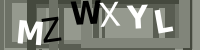# Formula Weight

In ceramics, this is the total weight of oxides in an oxide formula (a formula normally expresses the chemistry of a fired glaze, without the LOI present in the unfired raw materials). Since an oxide formula compares numbers of molecules (moles), the formula weight can be derived by simply summing the products of the oxide amounts and oxide weights.

When a formula is retotaled (e.g. to unify RO or R2O3), the formula weight changes accordingly. Thus the formula weight is not like an atomic weight, it is simply the weight of the formula as presented.

It is also possible, although much less practical, to express the chemistry of a material as an oxide formula. In this case, the formula weight includes the volatiles (e.g. non-ceramic oxides like CO2, CO, H2O, even pure carbon) that burn away during firing. Thus, a material with 10% weight loss on firing will have a formula weight that is 10% higher that for the ceramic oxides alone. The MDT (materials database) in the original Digitalfire desktop Insight used this technique. However. materials are much better expressed as a chemical analysis.

The formula weight of an oxide formula is not really something one needs to know. It never comes up in glaze chemistry except when one encounters a material whose makeup is expressed as a oxide formula. Knowing the weight is what enables deriving the LOI in the chemical analysis.

## Related Information

Glossary LOI Loss on Ignition is a number that appears on the data sheets of ceramic materials. It refers to the amount of weight the material loses as it decomposes to release water vapor and various gases during firing. Oxide Formula In ceramics, the chemistry of fired glazes is expressed as an oxide formula. There are direct links between the oxide chemistry and the fired physical properties. Substituting Materials by Weight: Why it does not work! Wollastonite is 50:50 CaO:SiO2. So why not just substitute 40 wollastonite for 20 calcium carbonate and 20 silica? The answer will help you see reason why we make such a big deal of glaze chemistry.

Name

Subject

Message